## Newton's Laws Applications Review

#### Navigate to:

Review Session Home - Topic Listing

Forces in 2 Dimensions - Home || Printable Version || Questions with Links

Answers to Questions:  All || #1-12 || #13-21 || #22-30

 [  #13 | #14 | #15 | #16 | #17 | #18 | #19 | #20 | #21  ]

### Part C: Force-Mass-Acceleration Relationships

Use the approximation that g= ~10 m/s2 to fill in the blanks in the following diagrams.

 13.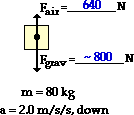Fgrav = m•g = ~800 N ∑Fy = may = (80 kg)•(2.0 m/s/s) ∑Fy = 160 N, down The Fgrav (down) and the Fair (up) must add up to 160 N, down. Thus, Fair must be smaller than Fgrav by 160 N. Fair = 640 N 14.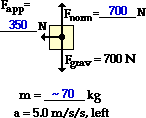Since  Fgrav = m•g, m can be calculated to be ~70 kg (m=Fgrav/g). Since ay = 0 m/s/s, Fnorm must equal Fgrav; so Fnorm = 700 N. ∑Fx = m•ax = (70 kg)•(5.0 m/s/s) ∑Fx = 350 N, left (Note that the ·Fx direction is always the same as the ax direction.) With Fapp being the only horizontal force, its value must be 350 N - the same as ·Fx. 15.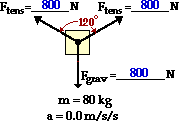Fgrav = m•g = ~800 N Since there are two forces pulling upwards and since the sign is hanging symmetrically, each force must supply an upwards pull equal to one-half the object's weight. So the vert pull (Fy) in each force is 400 N. The following triangle can be set up: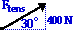Using trig, we can write: sin(30 deg.)=(400 N)/Ftens Solving for Ftens yields 800 N.

 16.A quick blank is Fgrav:  Fgrav = m•g = ~80 N Now resolve the 60-N force into components using trigonometry and the given angle measure: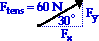Fx = 60 N•cos(30 deg) = 52 N Fy = 60 N•sin(30 deg) = 30 N Since the acceleration is horizontal, the sum of the vertical forces must equal 0 N. So Fgrav = Fy + Fnorm. Therefore Fnorm = Fgrav - Fy = 50 N. Knowing Fnorm and mu, the Ffrict can be determined: Ffrict = mu•Fnorm = 0.5*(50 N) = 25 N Now the horizontal forces can be summed: ∑Fx = Fx + Ffrict = 52 N, right + 25 N, left ∑Fx = 27 N, right Using Newton's second law, ∑Fx = m•ax So ax = (27 N)/(8 kg) = 3.4 m/s/s, right (3375... m/s/s) 17.The first step in an inclined plane problem is to resolve the weight vector into parallel and perpendicular components: Fpar = m•g•sin(angle) = (420 N)•sin(30 deg) = 210 N Fperp = m•g•cos(angle) = (420 N)•cos(30 deg) = 364 N The mass can be found as m = Fgrav/g m = Fgrav/g = (420 N)/(10 m/s/s) = ~42 kg The Fnorm acts opposite of and balances the Fperp. So Fnorm = Fperp = 364 N Knowing Fnorm and mu, the Ffrict can be determined: Ffrict = mu•Fnorm = 0.2*(364 N) = 73 N Now the forces parallel to the incline can be summed: ∑F|| = F|| + Ffrict = 210, down to left + 73 N, up to right ∑F|| = 137 N, down to left Using Newton's second law, ∑F|| = m•a|| So a|| = (137 N)/(42 kg) = 3.3 m/s/s 18.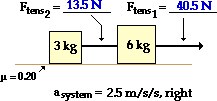Treating the two masses as a single system, it can be concluded that the net force on the 9-kg system is: ∑Fsystem = m•asystem = (9 kg)•(2.5 m/s/s) = 22.5 N, right The free-body diagram for the system is: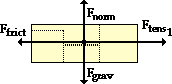The Fnorm supporting the 9-kg system is ~90 N. So the Ffrict acting upon the system is: Ffrict = mu• Fnorm = 0.20*(90 N) = 18 N, left So if ∑Fsystem = 22.5 N, right and Ffrict = 18 N, left, the rightward Ftens1 must equal 40.5 N. The Ftens2 force is found inside the system; as such it can not be determined through a system analysis. To determine the Ftens2, one of the individual masses must be isolated and a free-body analysis must be conducted for it. The 3-kg mass is selected and analyzed: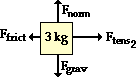The Fnorm and Fgrav balance each other; their value is ~30 N. The Ffrict on the 3-kg mass is: Ffrict = mu•Fnorm = 0.20*(30 N) = 6 N, left The net force on the 3-kg object is: ∑Fx = m•ax = (3 kg)•(2.5 m/s/s) = 7.5 N, right The horizontal forces must sum up to the net force on the 3-kg object; So ∑Fx = m•ax = Ftens2 (right) + Ffrict (left) 7.5 N, right = Ftens2 + 6 N, left The Ftens2 must be 13.5 N. 19.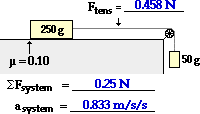Like most two-body problems involving pulleys, it is usually easiest to forgo the system analysis and conduct separate free-body analyses on the individual masses. Free-body diagrams, the chosen axes systems, and associated information is shown below.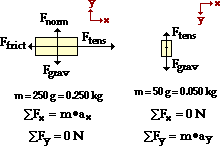Analyzing the Fx forces on the 250-g mass yields: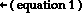max = Ftens - Ffrict Since Ffrict = mu•Fnorm and Fnorm = 2.5 N The Ffrict is (0.1)*(2.5 N) = 0.25 N. Substituting into equation 1 yields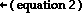(0.250 kg)•ax = Ftens - 0.25 N   Analyzing the Fy forces on the 50-g mass yields: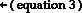may = Fgrav - Ftens Substituting m and Fgrav values into equation 3 yeilds: (0.050 kg)*ay = (0.500 N) - Ftens The above equation can be rearranged to: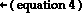Ftens = (0.500 N) - (0.050 kg)*ay Equation 4 provides an expression for Ftens; this can be substituted into equation 2: (0.250 kg)•ax = (0.500 N) - (0.050 kg)*ay - 0.25 N Now since both masses accelerate at the same rate, ax =ay and the above equation can be simplified into an equation with 1 unknown - the acceleration (a): (0.250 kg)•a = (0.500 N) - (0.050 kg)*a - 0.25 N After a few algebra steps, the acceleration can be found: (0.0300 kg)•a = 0.25 N a = 0.833 m/s/s Now that a has been found, its value can be substituted back into equation 4 in order to solve for Ftens: Ftens = (0.500 N) - (0.050 kg)*(0.833 m/s/s) Ftens = 0.458 N 20.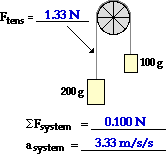This problem can most easily be solved using separate free-body analyses on the individual masses. Free-body diagrams, the chosen axes systems, and associated information is shown below.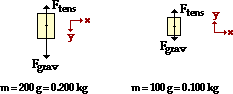Note that the positive y-axis is chosen as being downards on the 200-g mass since that is the direction of its acceleration. Similarly, it chosen as upwards on the 100-g mass since that is the direction of its acceleration. For the 200-gram mass, the sum of the vertical forces equals the mass times the acceleration:  Fgrav - Ftens = m•ay2.00 N - Ftens = (0.200 kg)•ay The same type of analysis can be conducted for the 100-gram mass: Ftens - Fgrav = m•ayFtens - 1.00 N = (0.100 kg)•ay Equation 2 can be rearranged to obtain an expression for the tension force:Ftens = (0.100 kg)•ay + 1.00 N This expression for Ftens can be substituted into equation 1 in order to obtain a single equation with acceleration (ay) as the unknown. The ay value can be solved for. 2.00 N -[(0.100 kg)•ay + 1.00 N] = (0.200 kg)•ay 2.00 N - 1.00 N = (0.200 kg)•ay + (0.100 kg)•ay 1.00 N = (0.300 kg)•ay ay = (1.00 N)/(0.300 kg) = 3.33 m/s/s Now with ay known, its value can be substituted into equation 3 in order to determine the tension force: Ftens = (0.100 kg)•ay + 1.00 N Ftens = (0.100 kg)•(3.33 m/s/s) + 1.00 N Ftens = 0.333 N + 1.00 N = 1.33 N 21.Like #20, this problem can most easily be solved using separate free-body analyses on the individual masses. Free-body diagrams, the chosen axes systems, and associated information is shown below. Note that in chosing the axis system, it has been assumed that object 1 will accelerate up the hill and object 2 will accelerate downwards. If this ends up to be false, then the acceleration values will turn out to be negative values.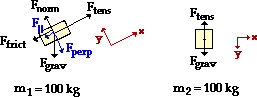Object 1 is on an inclined plane. The usual circumstances apply; their is no acceleration along what has been designated as the y-axis. Fnorm = Fperp = m•g•cos(theta) = 888.2 N The parallel component of Fgrav is F|| = m•g•sin(theta) = (100 kg)•(9.8 m/s2)•sin(25) F|| = 414.2 N The Ffrict value can be found from the Fnorm value: Ffrict = mu•Fnorm = (0.35)•(888.2 N) = 310.9 N The ∑Fx = m•ax equation can now be written: ∑Fx = m•ax Ftens - Ffrict - F|| = m•axFtens - 310.9 N - 414.2 N = m•ax (Note that the Ffrict and F|| forces are subtracted from Ftens since they are heading in the direction of the negative x-axis.) The above process can be repeated for object 2. The ∑Fy = m•ay equation can now be written: ∑Fy = m•ay Fgrav - Ftens = m•ay(980 N) - Ftens = m•ay The separate free-body analyses have provided two equations with two unknowns; the task at hand is to use these two equations to solve for Ftens and a. Equation 2 can be re-written as (980 N) - m•ay = Ftens Since both objects accelerate together at the same rate, the ax for object 1 is equal to the ay value for object 2. The subscripts x and y can be dropped and a can be inserted into each equation.(980 N) - m•a = Ftens Equation 3 provides an expression for Ftens in terms of a. This expression is inserted into equation 1 in order to solve for acceleration. The steps are shown below. (980 N) - m•a - 310.9 N - 414.2 N = m•a = 2•m•a 254.9 N = 2•(100 kg)•a 1.27 m/s2 = a The value of a can be re-inserted into equation 3 in order to solve for Ftens: Ftens = (980 N) - m•a =(980 N) - (100 kg)•(1.27 m/s2) Ftens = 853 N

 Useful Web Links Finding Acceleration || Net Force Problems Revisited || Inclined Planes

#13 | #14 | #15 | #16 | #17 | #18 | #19 | #20 | #21  ]

#### Navigate to:

Review Session Home - Topic Listing

Forces in 2 Dimensions - Home || Printable Version || Questions with Links

Answers to Questions:  All || #1-12 || #13-21 || #22-30

## You Might Also Like ...

Users of The Review Session are often looking for learning resources that provide them with practice and review opportunities that include built-in feedback and instruction. If that is what you're looking for, then you might also like the following:

The Calculator Pad includes physics word problems organized by topic. Each problem is accompanied by a pop-up answer and an audio file that explains the details of how to approach and solve the problem. It's a perfect resource for those wishing to improve their problem-solving skills.

Visit: The Calculator Pad Home | Calculator Pad - Forces in Two Dimensions

2. Minds On Physics the App Series

Minds On Physics the App ("MOP the App") is a series of interactive questioning modules for the student that is serious about improving their conceptual understanding of physics. Each module of the series covers a different topic and is further broken down into sub-topics. A "MOP experience" will provide a learner with challenging questions, feedback, and question-specific help in the context of a game-like environment. It is available for phones, tablets, Chromebooks, and Macintosh computers. It's a perfect resource for those wishing to refine their conceptual reasoning abilities. Part 2 of the series includes Forces in Two Dimensions.

Visit: MOP the App Home || MOP the App - Part 2